##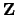is Noetherian

The ringof integers is Noetherian because every ideal ofis generated by one element.

Proposition 4.1.9   Every ideal of the ringof integers is principal.

Proof. Suppose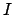is a nonzero ideal in. Let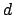the least positive element of. Suppose that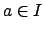is any nonzero element of. Using the division algorithm, write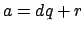, where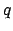is an integer and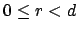. We have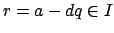and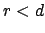, so our assumption thatis minimal implies that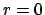, so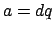is in the ideal generated by. Thusis the principal ideal generated by.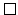Proposition 4.1.6 and 4.1.9 together imply that any finitely generated abelian group is Noetherian. This means that subgroups of finitely generated abelian groups are finitely generated, which provides the missing step in our proof of the structure theorem for finitely generated abelian groups.

William Stein 2004-05-06